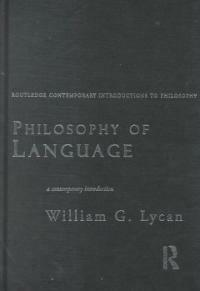> 상세정보

상세정보Philosophy of language : a contemporary introduction (24회 대출)

자료유형
단행본
개인저자
Lycan, William G.
서명 / 저자사항
Philosophy of language : a contemporary introduction / William G. Lycan.
발행사항
London ;   New York :   Routledge,   2000.
형태사항
xiv, 243 p. : ill. ; 25 cm.
총서사항
Routledge contemporary introductions to philosophy
ISBN
0415171156 (hb.) 0415171164 (pbk.)
서지주기
Includes bibliographical references (p. -238) and index.
일반주제명
Language and languages --Philosophy.
 000 00000cam u2200205 a 4500 001 000000694624 005 20210826102745 008 990409s2000 enka b 001 0 eng 010 ▼a 99029547 020 ▼a 0415171156 (hb.) 020 ▼a 0415171164 (pbk.) 040 ▼a DLC ▼c DLC ▼d 211009 042 ▼a pcc 049 1 ▼l 111177308 050 0 0 ▼a P106 ▼b .L886 2000 082 0 4 ▼a 401 ▼2 23 084 ▼a 401 ▼2 DDCK 090 ▼a 401 ▼b L981p 100 1 ▼a Lycan, William G. 245 1 0 ▼a Philosophy of language : ▼b a contemporary introduction / ▼c William G. Lycan. 260 ▼a London ; ▼a New York : ▼b Routledge, ▼c 2000. 300 ▼a xiv, 243 p. : ▼b ill. ; ▼c 25 cm. 490 1 ▼a Routledge contemporary introductions to philosophy 504 ▼a Includes bibliographical references (p. -238) and index. 650 0 ▼a Language and languages ▼x Philosophy. 830 0 ▼a Routledge contemporary introductions to philosophy.

소장정보

No. 소장처 청구기호 등록번호 도서상태 반납예정일 예약 서비스
No. 1 소장처 청구기호 401 L981p 등록번호 111177308 도서상태 대출가능 반납예정일 예약 서비스
No. 2 소장처 청구기호 401 L981p 등록번호 511048279 도서상태 대출가능 반납예정일 예약 서비스

컨텐츠정보

목차

CONTENTS

Preface = xiii

Acknowledgements = xv

Chapter 1 : Introduction : meaning and reference = 1

Overview = 2

Meaning and understanding = 3

The Referential Theory = 4

Summary = 8

Questions = 8

Notes = 8

PARTⅠ : REFERENCE AND REFERRING = 9

Chapter 2 : Definite descriptions = 11

Overview = 12

Singular terms = 13

Russell's Theory of Descriptions = i6

objections to russell's theory = 21

Donnellan's distinction = 26

Anaphora = 31

Summary = 32

Questions = 33

Notes = 33

Chapter 3 : Proper names : the Description Theory = 35

Overview = 36

Russell's Name Claim = 37

Opening objections = 40

Searle's "Cluster Theory' = 42

Kripke's critique = 43

Summary = 48

Questions = 48

Notes = 48

Chapter 4 : Proper names : Direct Reference and the Causal-Historical Theory = 50

Overview = 51

Possible worlds = 52

Rigidity and proper names = 53

Direct Reference = 55

The Causal-Historical Theory = 60

Problems for the Causal-Historical Theory = 62

Natural-kind terms and "Twin Earth" = 66

Summary = 68

Questions = 69

Notes = 69

PART Ⅱ : THEORIES OF MEANING = 73

Chapter 5 : Traditional theories of meaning = 75

Overview = 76

Ideational theories = 78

The Proposition Theory = 80

Summary = 86

Questions = 87

Notes = 87

Chapter 6 : " Use" theories = 88

Overview = 89

"Use" in a roughly Wittgensteinian sense = 90

Objections and some replies = 93

Summary = 98

Questions = 98

Notes = 98

Chapter 7 : Psychological theories : Grice's program = 100

Overview = 101

Grice's basic idea = 102

Speaker-meaning = 103

Sentence meaning = 108

Summary = 113

Questions = 113

Notes = 113

Chapter 8 : Verificationism = 115

Overview = 116

The theory and its motivation = 117

Some objections = 119

The big one = 124

Two Quinean issues = 125

Summary = 127

Questions = 127

Notes = 128

Chapter 9 : Truth-Condition Theories : Davidson's program = 129

Overview = 130

Truth conditions = 131

Truth-defining natural languages = 136

Initial objections = 140

Summary = 146

Questions = 147

Notes = 147

Chapter 10 : Truth-Condition Theories : possible worlds and intensional semantics = 149

Overview = 150

Truth conditions reconceived = 151

Advantages over Davidson's view = 154

Remaining objections = 156

Summary = 158

Questions = 158

Notes = 159

PART Ⅲ : PRAGMATICS AND SPEECH ACTS = 161

Chapter 11 : Semantic pragmatics = 163

Overview = 164

Semantic vs pragmatic pragmatics = 165

The problem of deixis = 166

The work of semantic pragmatics = 169

Summary = 171

Questions = 171

Notes = 171

Chapter 12 : Speech acts and illocutionary force = 173

Overview = 174

Performatives = 175

Rules and infelicities = 176

Force, content, and perlocution = 178

Cohen's problem = 181

Summary = 184

Questions = 185

Notes = 185

Chapter 13 : Implicative relations = 187

Overview = 188

Conveyed meanings and Invited inferences = 189

Conversational implicature = 191

"Presupposition" and conventional implicature = 195

Indirect force

Summary = 202

Questions = 202

Notes = 203

PART Ⅳ THE DARK S1DE = 205

Chapter 14 : Metaphor = 207

Overview = 208

A philosophical b1as = 209

The issues, and two simple theories = 210

The Figurative S1mile Theory = 214

The Pragmatic Theory = 217

Metaphor as analogical = 722

Summary = 224

Quest1ons = 224

Notes = 225

Glossary = 227

Bibliography = 229

Index = 239

관련분야 신착자료

파롤

Gusdorf, Georges (2021)

남기춘 (2021)

How languages are learned / 5th ed

Lightbown, Patsy (2021)

Formal semantics : a mathematical approach to the analysis of meaning

Song, Mean-young (2021)

(알아두면 쓸모 있는) 어원잡학사전 : 평범한 단어들의 특별한 어원이야기

Foote, Patrick (2021)

L'intelligence artificielle des textes : des algorithmes à l'interprétation

Mayaffre, Damon (2021)

홍승우 (2021)

The handbook of speech perception / 2nd ed

Pardo, Jennifer S. (2021)